##### Statistics Workbook For Dummies with Online Practice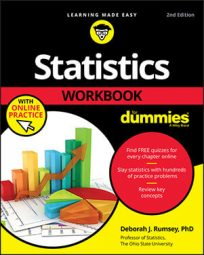Statistics and math are very different subjects, but you use a certain amount of mathematical tools to do statistical calculations. Sometimes you can understand the statistical idea but get bogged down in the formulas and calculations and end up getting the wrong answer. Avoid making the common math mistakes that can cost you points on homework and exams. Read on to increase your confidence with the math tools you need for statistics.©WAYHOME Studio/Shutterstock.com

The most basic math symbols are +, –, ∙ (multiplication), and / (division); but have you ever seen the following sign?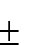It means plus or minus and indicates a lower bound and an upper bound for your answer. Other commonly used math symbols involve the Greek letter “capital” sigma, which stands for summation.

In math formulas, you often leave out the multiplication sign; for example, 2x means 2 × x.

If you come across a math symbol that you don’t understand, ask for help. You can never get comfortable with that symbol until you know exactly what you use it for and why. You may be surprised that after you lift the mystique, math symbols aren’t really as hard as they seem to be. They simply provide you with a shorthand way of expressing something that you need to do.

## Uproot roots and powers

Remember that squaring a number means multiplying it by itself two times, not multiplying by two. And taking the square root means finding the number whose square gives you your result; it doesn’t mean dividing the number by 2. Using math notation, x2 means square the value (so for x = 3, you have 32 = 9); and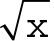means take the square root (for x = 9, this means the square root of 9 is 3).

You can’t take the square root of a negative number, because you can’t square anything to get a negative number back. So, anything under a square root sign has to be a nonnegative quantity (that is, it has to be greater than or equal to 0).

These ideas may seem straightforward, but like everything else, they can get complex very fast. If you need to find the square root of an entire expression, put everything under the square root sign in parentheses so your calculator knows to take the square root of the entire expression, not just part of it.

Statistics often deal with percentages — numbers that in decimal form are between 0 and 1. You need to know that numbers between 0 and 1 often act differently than large numbers do. For example, numbers larger than 1 get smaller when you take the square root, but numbers between 0 and 1 get larger when you take the square root. For example, the square root of 4 is 2 (which is smaller than 4), but the square root of 1/4 is 1/2 (which is larger). And when you take powers, the opposite happens. Numbers larger than 1 that you square get larger; for example, 3 squared is 9 (which is larger than 3). Numbers between 0 and 1 that you square get smaller; for example, 1/3 squared is 1/9 (which is smaller).

## Treat fractions with extra care

Every fraction contains a top (numerator) and a bottom (denominator). For example, in the fraction 3/7, 3 is the numerator and 7 is the denominator. But what does a fraction really mean? It means division. The fraction 3/7 means take the number 3 and divide it by 7.

A common mistake is to read fractions upside down in terms of what you divide by what. The fraction 1/10 means 1 divided by 10, not 10 divided by 1. If you can hold on to an example like this that you know is correct, it can stop you from making this mistake again later when the formulas get more complicated.

## Obey the order of operations

To follow the order of math operations, remember “PEMDAS”: Parentheses, Exponents (powers of a number), Multiplication and Division (interchangeable), and Addition and Subtraction. Failing to follow the order of operations can result in a big mistake.

To remember the letters in PEMDAS for the order of operations, try this: “Please Excuse My Dear Aunt Sally.”

Suppose, for example, that you need to calculate the following: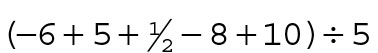.

First, calculate what’s in parentheses. You can either type it just as it looks into your calculator or do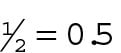separately and then plug it in as –6 + 5 + 0.5 – 8 + 10. You should get 3/2 or 1.5. Next, divide by 5 to get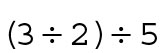or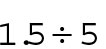which equals 0.3.

## Avoid rounding errors

Rounding errors can seem small, but they can really add up — literally. Many statistical formulas contain several different types of operations that you can do either all at once, using parentheses properly, or separately, as many students elect to do. Doing the operations separately and writing them down with each step is fine, as long as you don’t round off numbers too much at each stage.

For example, suppose that you have to calculate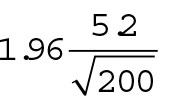You want to write down each step separately rather than calculate the equation all at once. Suppose that you round off to one digit after the decimal point on each calculation. First, you take the square root of 200 (which rounds to 14.1), and then you take 5.2 divided by 14.1, which is 0.369; you round this to 0.4. Next, you take 1.96 times 0.4 to get 0.784, which you round to 0.8. The actual answer, if you do all the calculations at once with no rounding, is 0.72068, which safely rounds to 0.72. What a huge difference! What would this difference cost you on an exam? At worst, your professor would reject your answer outright, because it strays too far from the correct one. At best, he would take off some points, because your answer isn’t precise enough.

Instead of rounding to one digit after the decimal point, suppose that you round to two digits after the decimal point each time. This still gives you the incorrect answer of 0.73. You’ve come closer to the correct answer, but you’re still technically off, and points may be lost. Statistics is a quantitative field, and teachers expect precise answers. What should you do if you want to do calculation steps separately? Keep at least two significant digits after the decimal point during each step, and at the very end, round off to two digits after the decimal point.

Don’t round off too much too soon, especially in formulas where many calculations are involved. Your best bet is to use parentheses and use all the decimal places in your calculator. Otherwise, keep at least two significant digits after the decimal point until the very end.

## Get comfortable with statistical formulas

Don’t let basic math and statistical formulas get in your way. Think of them as mathematical shorthand. Suppose that you want to find the average of some numbers. You sum the numbers and divide by n (the size of your data set). If you have only a few numbers, writing out all the instructions is easy, but what if you have 1,000 numbers? Mathematicians have come up with formulas as a way of saying quickly what they want you to do, and the formulas work no matter the size of your data set. The key is getting familiar with formulas and practicing them.

## Stay calm when formulas get tough

Suppose that you encounter a formula that’s a little complicated? How do you remain calm and cool? By starting with small formulas, learning the ropes, and then applying the same rules to the bigger formulas. That’s why you need to understand how the “easy” formulas work and be able use them as formulas; you shouldn’t just figure them out in your head, because you don’t need the formula in that case. The easy formulas build your skills for when things get tougher.

Many times in math and statistics, different variables are related to each other. For example, to get the area of a square, you take the length of one of the sides and multiply it by itself. In mathematical notation, the formula looks like this: A = s2. This formula really represents a function. It says that the area of the square depends on the length of its sides. It also means that all you have to know is the length of one of the sides to get the area of the square. In math jargon, you say that the area of a square is a function of the length of its sides. Function just means “depends on.”

Suppose that you have a line with the equation y = 2x + 3. The equation conveys that x and y are related, and you know how they’re related. If you take any value of x, multiply it by two and add three, you get the corresponding value for y. Suppose that you want to find y when x is –2. To find y for a given x, plug in that number for x and simplify it. In this case, you have y = (2)(–2) + 3. This simplifies to y = –4 + 3 = –1.

You can also take this same function and plug in any value for y to get its corresponding value for x. For example, suppose that you have y = 2x + 3, and you’re given y = 4 and asked to solve for x. Plugging in 4 for y, you get 4 = 2x + 3. The only difference is, you normally see the unknown on one side of the equation and the number part on the other. In this case, you see it the other way around. Don’t worry about how it looks; remember what you need to do. You need to get x alone on one side, so use your algebra skills to make that happen. In this case, subtract 3 from each side to get 4 – 3 = 2x, or 1 = 2x. Now divide each side by 2 to get 0.5 = x. You have your answer.

You can use a formula in many different ways. If you have all the other pieces of information, you can always solve for the remaining part, no matter where it sits in the equation. Just keep your cool and use your algebra skills to get it done.

Certain commonly used functions have names. For example, an equation that has one x and one y is called a linear function, because when you graph it, you get a straight line. Statistics uses lines often, and you need to know the two major parts of a line: the slope and the y-intercept. If the equation of the line is in the form y = mx + b, m is the slope (the change in y over change in x), and b is the y-intercept (the place where the line crosses the y-axis). Suppose that you have a line with the equation y = –2x – 10. In this case, the y-intercept is –10, and the slope is–2.

The slope is the number in front of the x in the equation y = mx + b. If you rewrite the previous equation as y = –10 – 2x, the slope is still–2, because –2 is the number that goes with the x. And –10 is still the y-intercept.

You should always look at your answer to see whether it makes sense, in terms of what kind of number you expect to get. Can the number you’re calculating be negative? Can it be a large number or a fraction? Does this number make sense? All these questions can help you catch mistakes on exams and homework before your instructor does.

In any fraction, if the numerator (top) is larger than the denominator (bottom), the result is greater than 1. If the numerator (top) is smaller than the denominator (bottom), the result is less than 1. And if the numerator (top) and denominator (bottom) are exactly equal, the result is exactly 1.

You see the instructions “Show your work!” on your exams, and your instructor harps and harps on it, but still, you don’t quite believe that showing your work can be that important. Take it from a seasoned professor, it is. Here’s why:
• Showing your work helps the person grading your paper see exactly what you tried to do, even if the answer is wrong. This works to your advantage if your work was on the right track. The only way to get partial credit for your work is to show that you had the right idea, and you must do this in writing.
• Not showing your work makes it hard on the person grading your paper and can cost you points in an indirect way. Grading is a tremendous amount of work. Here's how the “grading effect” on your teacher ultimately affects you. Your teacher has a big pile of papers to grade and only so much time (and energy) to grade them all. A paper with a big messed up area of scribbling, erasing, crossing out, and smudging rears its ugly head. It has no clear tracks as to what’s happening or what the student was thinking. Numbers are pushed around every which way with no clear-cut steps or pattern to follow. How much time can (will) teachers spend trying to figure out this problem? Teachers have to move on at some point; we can only do so much to try to figure out what students were thinking during an exam.

Here’s another typical situation. A teacher looks at two papers, both with the right answer. One person wrote out all the steps, labeled everything, and circled the answer, but the other person simply wrote down the answer. Do you give both people full credit? Some teachers do, but many don’t. Why? Because the instructor isn’t sure whether you did the work yourself. Teachers don’t typically advocate doing math “in your head.” We want you to show your work, because someday, even for you, the formulas will get so complicated that you can’t rely on your mind alone to solve them. Plus, you do need to show evidence that the work is your own.

What if you write down the answer, and the answer is wrong, but only a tiny little mistake led to the error? With no tracks to show what you were thinking, the teacher can’t give you partial credit, and the littlest of mistakes can cost you big time.

• Showing your work establishes good habits that last a lifetime. Each time you work a problem, whether you’re working in class, on homework, to study for an exam, or on an exam, if you follow the same procedure each time, good things will happen.

Here’s a great way to work a math-related statistics problem:

1. Write out the formula you plan to use, in its entirety (letters included).
2. Clearly write down what number you plug in for each variable in the formulas; for example, x = 2 and y = 6.
3. Work out the calculations in a step-by-step manner, showing each step clearly.